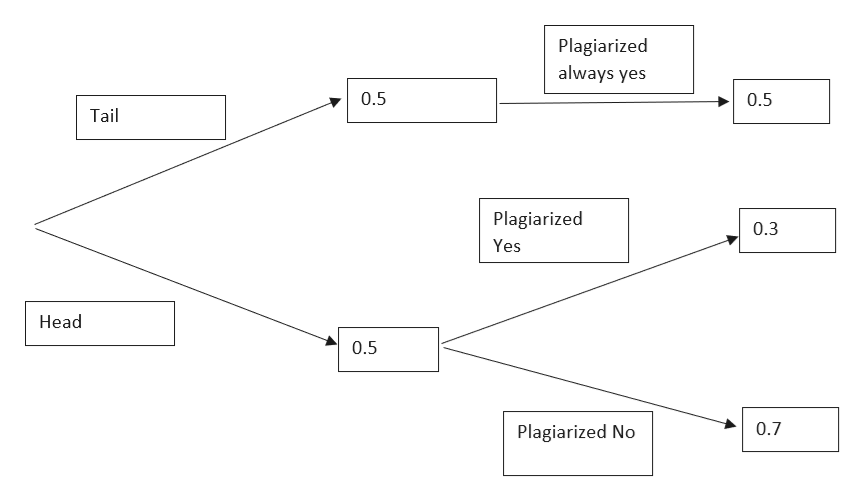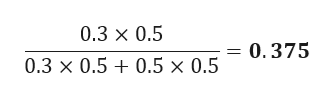We ask each student being surveyed to toss a coin in private. If the coin lands "heads," they are to answer the question, "Have you plagiarized a term paper?" truthfully. If the coin lands "tails," they are told always to answer "yes," whether they have in fact plagiarized or not. Imagine that the true fraction of students who have plagiarized is in fact 0.3, and imagine that participants in the survey indeed follow the randomized response procedure accurately and honestly. What is the conditional probability that a randomly chosen student who answers "yes" to the survey has in fact plagiarized?

Question

We ask each student being surveyed
to toss a coin in private. If the coin lands "heads," they are to answer the question, "Have you plagiarized a term paper?" truthfully. If the coin lands "tails," they are told always to answer "yes," whether they have in fact plagiarized or not. Imagine that the true fraction of
students who have plagiarized is in fact 0.3, and imagine that participants in the survey
indeed follow the randomized response procedure accurately and honestly. What is the
conditional probability that a randomly chosen student who answers "yes" to the survey
has in fact plagiarized?

Step 1

Given data
The response is always yes when tail occurs
Making tree diagram as per the questionhelp_outlineImage TranscriptionclosePlagiarized always yes 0.5 0.5 Tail Plagiarized 0.3 Yes Head 0.5 0.7 Plagiarized No fullscreen
Step 2

conditional probability that a randomly chosen student who answers ...help_outlineImage Transcriptionclose0.3 x 0.5 = 0.375 0.3 x 0.50.5 x 0.5 fullscreen

Want to see the full answer?

See Solution

Want to see this answer and more?

Our solutions are written by experts, many with advanced degrees, and available 24/7

See Solution
Tagged in

Basic Probability